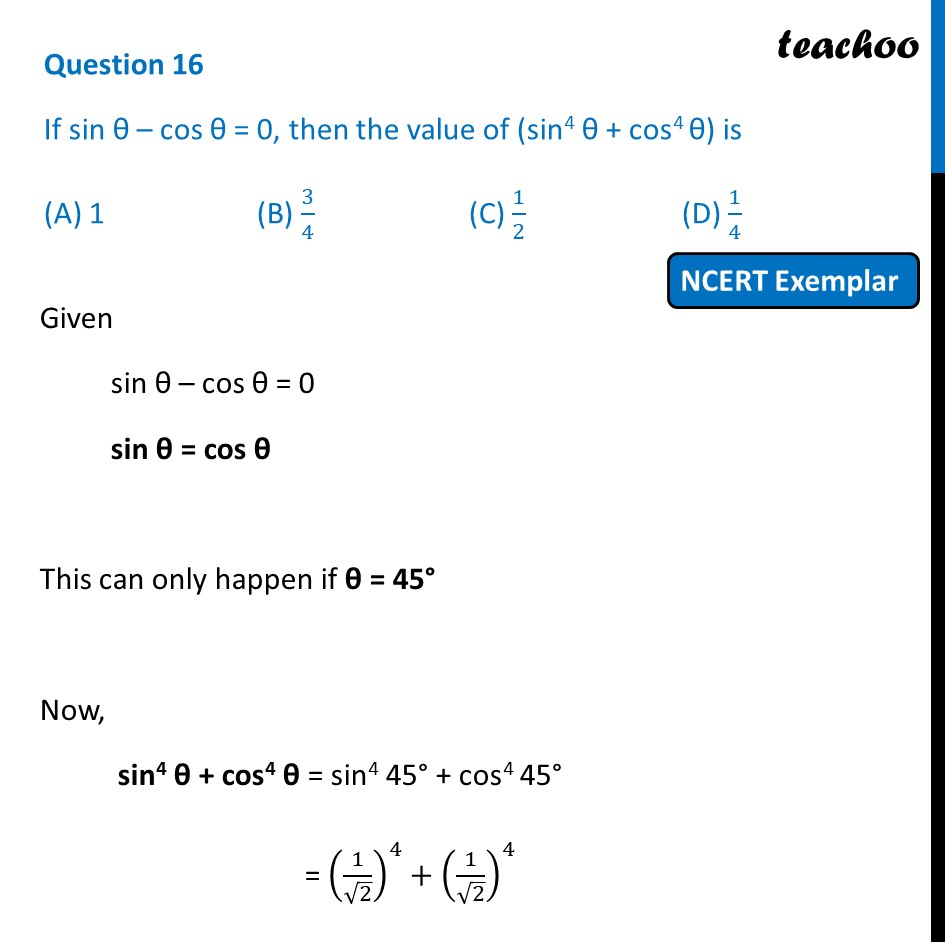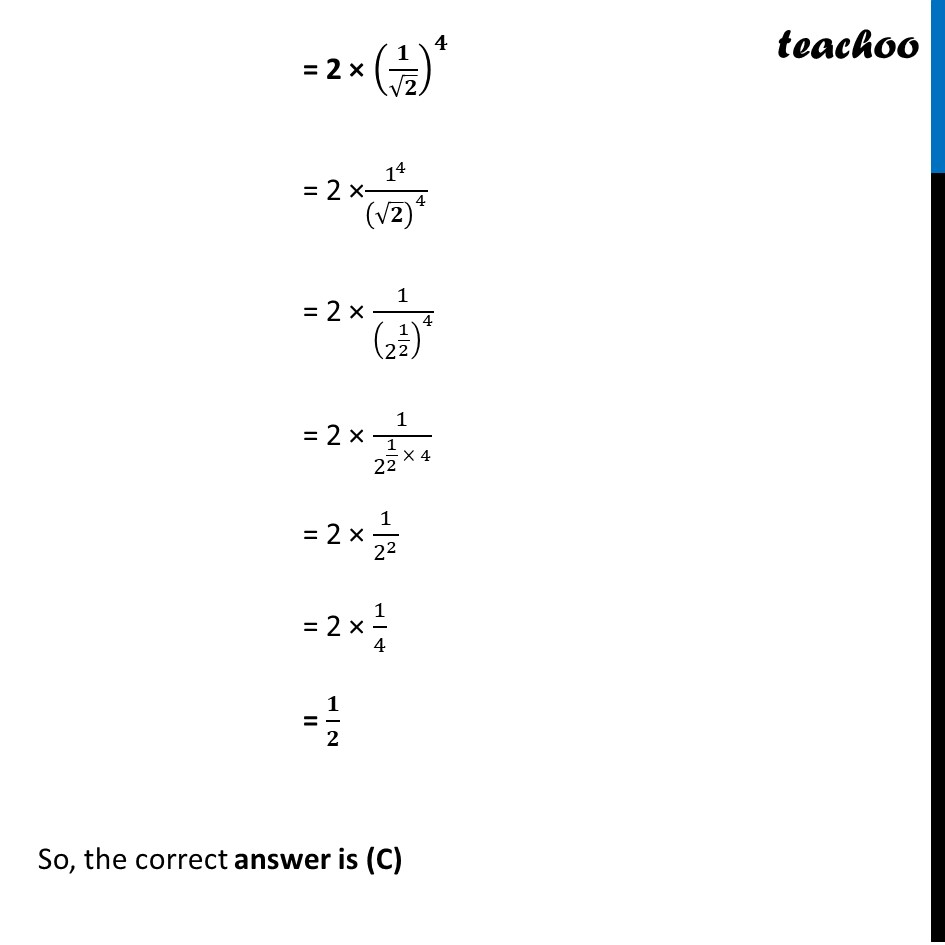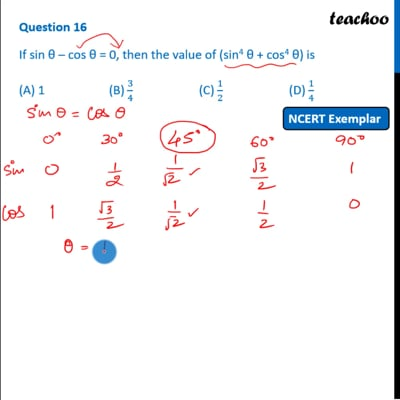NCERT Exemplar - MCQ

Chapter 8 Class 10 Introduction to Trignometry
Serial order wise

## (D) 1/4This video is only available for Teachoo black users

Introducing your new favourite teacher - Teachoo Black, at only ₹83 per month

### Transcript

Question 16 If sin θ – cos θ = 0, then the value of (sin4 θ + cos4 θ) is (A) 1 (B) 3/4 (C) 1/2 (D) 1/4 Given sin θ – cos θ = 0 sin θ = cos θ This can only happen if θ = 45° Now, sin4 θ + cos4 θ = sin4 45° + cos4 45° = (1/√2)^4+(1/√2)^4 = 2 × (𝟏/√𝟐)^𝟒 = 2 × [(1/√2)^2 ]^2 = 2 × [1/2]^2 = 2 × 1/4 = 𝟏/𝟐 So, the correct answer is (C)